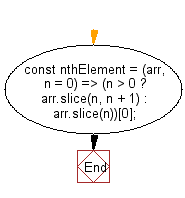# JavaScript : Get the nth element of a given array

## JavaScript fundamental (ES6 Syntax): Exercise-109 with Solution

Write a JavaScript program to get the nth element of a given array.

• Use Array.prototype.slice() to get an array containing the nth element at the first place.
• If the index is out of bounds, return undefined.
• Omit the second argument, n, to get the first element of the array.

Sample Solution:

JavaScript Code:

``````//#Source https://bit.ly/2neWfJ2
const nthElement = (arr, n = 0) => (n > 0 ? arr.slice(n, n + 1) : arr.slice(n));
console.log(nthElement(['a', 'b', 'c'], 1));
console.log(nthElement(['a', 'b', 'b'], -3));
```
```

Sample Output:

```b
a
```

Pictorial Presentation:Flowchart:Live Demo:

See the Pen javascript-basic-exercise-109-1 by w3resource (@w3resource) on CodePen.

Improve this sample solution and post your code through Disqus

What is the difficulty level of this exercise?

Test your Programming skills with w3resource's quiz.

﻿

## JavaScript: Tips of the Day

Chunks an array into n smaller arrays

Example:

```const tips_chunkIntoN = (arr, n) => {
const size = Math.ceil(arr.length / n);
return Array.from({ length: n }, (v, i) =>
arr.slice(i * size, i * size + size)
);
}
console.log(tips_chunkIntoN([1, 2, 3, 4, 5, 6, 7,8], 4));
```

Output:

```[[1,2],[3,4],[5,6],[7,8]]
```